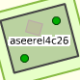# Tile coordinates from lat+lon+zoom formula problem?

 0 Hi All, I'm trying to get tile coordinates of lat/lon points on various zoom level maps using the code shown in the OSM wiki. I started testing with the following python code from the OSM wiki: -- code ------------------ ``````import math def deg2num(latdeg, londeg, zoom): latrad = math.radians(latdeg) n = 2.0 ** zoom xtile = int((londeg + 180.0) / 360.0 * n) ytile = int((1.0 - math.log(math.tan(latrad) + (1 / math.cos(latrad))) / math.pi) / 2.0 * n) return (xtile, ytile) zoom = 4 lon = 0.0 print 'zoom=%d, lon=%.1f' % (zoom, lon) for lat in range(80,91): print 'lat=%.2f, result=%s' % (lat, str(deg2num(lat, lon, zoom))) `````` -- end code -------------- This code, when run, shows this: ``````zoom=4, lon=0.0 lat=80.00, result=(8, 1) lat=81.00, result=(8, 1) lat=82.00, result=(8, 1) lat=83.00, result=(8, 0) lat=84.00, result=(8, 0) lat=85.00, result=(8, 0) lat=86.00, result=(8, 0) lat=87.00, result=(8, -1) lat=88.00, result=(8, -2) lat=89.00, result=(8, -4) lat=90.00, result=(8, -88) `````` Note that the values for latitudes greater than 86.0 degrees return NEGATIVE tile coordinates. I don't see any indication that the OSM formula is valid only if abs(lat) <= 85 degrees, so what am I doing wrong? Or is this a known limitation of the geomapping? asked 19 Oct '14, 07:39rzzzwilson 1●1●1●1 accept rate: 0%aseerel4c26 ♦ 32.5k●18●244●554

 4 See https://en.wikipedia.org/wiki/Mercator_projection you will need to use a different projection if you want to display the pole regions. answered 19 Oct '14, 10:12SimonPoole ♦ 42.8k●13●313●673 accept rate: 19%
 toggle preview community wiki:

By Email:

Markdown Basics

• *italic* or _italic_
• **bold** or __bold__
• image?![alt text](/path/img.jpg "title")
• numbered list: 1. Foo 2. Bar
• to add a line break simply add two spaces to where you would like the new line to be.
• basic HTML tags are also supported

Question tags:

×268
×138
×72

question asked: 19 Oct '14, 07:39

question was seen: 4,937 times

last updated: 19 Oct '14, 12:10